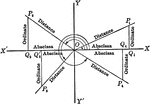### Reference Angles/Triangles Formed by Angles in Quadrants With Labels

Lines drawn to horizontal to form triangle ratios (reference triangles, angles). Axes, quadrants, abscissa,…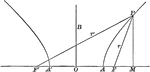### Point on a Hyperbola

Illustration of a point on a hyperbola. "If d denotes the abscissa (x-coordinate) of a point of an hyperbola,…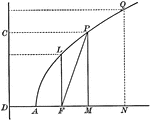### Parabola With Coordinates and Latus Rectum

Illustration of a parabola showing that any point of a parabola is the mean proportional between the…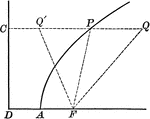### Point on Parabola

Illustration of a parabola showing point P is equidistant to the focus and the directrix.### Point on Parabola

Illustration of a parabola. "If a line PT is drawn from any point P of the curve, bisecting the angle…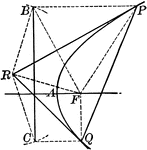### Tangent to a Parabola

Illustration of a tangent line drawn from an external point to a parabola.